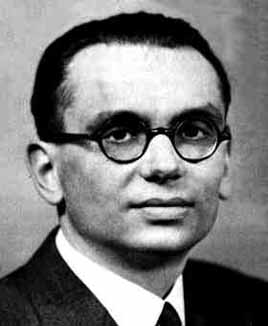Kurt Gödel (1906 – 1978) was an Austrian-American logician, mathematician and philosopher.

In 1931, Gödel published his two incompleteness theorems.

1. If the system is consistent, it cannot be complete.

2. The consistency of the axioms cannot be proved within the system.

Within some limitations the first theorem means that some Mathematical statements which are true cannot be proved to be true. That means that some mathematician may be trying to prove statements which are impossible to prove.

Again within some limitations, the second theorem brought an end to the efforts of Hilbert, Frege, Russell etc. to justify the foundations of Mathematics as it states that to do so is impossible.

One of the Famous People on this site